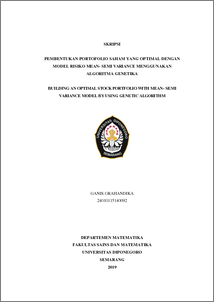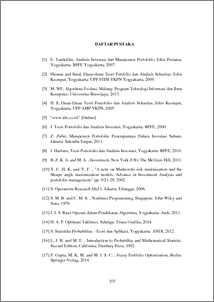# BUILDING AN OPTIMAL STOCK PORTFOLIO WITH MEAN- SEMI VARIANCE MODEL BY USING GENETIC ALGORITHM

Grahandika, Ganis (2019) BUILDING AN OPTIMAL STOCK PORTFOLIO WITH MEAN- SEMI VARIANCE MODEL BY USING GENETIC ALGORITHM. Undergraduate thesis, UNDIP.Preview
PDF
460KbPDF
Restricted to Repository staff only

1937KbPreview
PDF
59Kb

## Abstract

Genetic algorithm is a metaheuristic search algorithm based on the mechanism of natural selection and genetic operations to get a solution. The solution obtained from the genetic algorithm is determined by the operator and the parameters used. One of the problems that can be solved using genetic algorithms is the formation of an optimal stock portfolio. In the formation of stock portfolios, the portfolio model is also used, the mean semi variance, to calculate the level of profit and loss level that exists in the period May 2017 - December 2018. The shares used are LQ 45 shares with each share grouped in a certain group of shares totaling 8. The 8 stocks are then searched for the optimal weight of each share by using a genetic algorithm. The conventional method which will be used as the basis for choosing the optimal stock portfolio formation decision is by manual calculation using a lingo application with a mean semi variance risk model. From the calculation results, it is found that solving the problem of the formation of an optimal stock portfolio using genetic algorithms provides optimal solution results. The results obtained are by using a crossover operator in the form of extended intermediate crossover, mutation using random mutation, and selection using selection elistism. From these operators, optimal results were obtained with a population of 100, generation of 275, and a crossover rate of 0.6 mutation rate of 0.4. Keywords: Genetic Algorithms, Stock Portfolios, Mean Semi Variance, Optimal, Stocks.

Item Type: Thesis (Undergraduate) Q Science > QA Mathematics Faculty of Science and Mathematics > Department of Mathematics 84235 INVALID USER 12 Jun 2022 12:34 12 Jun 2022 12:34

Repository Staff Only: item control page